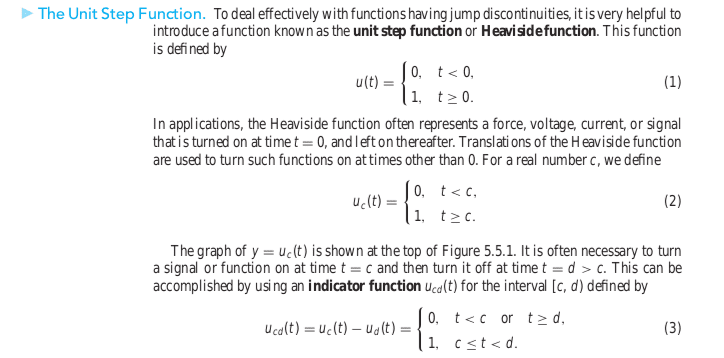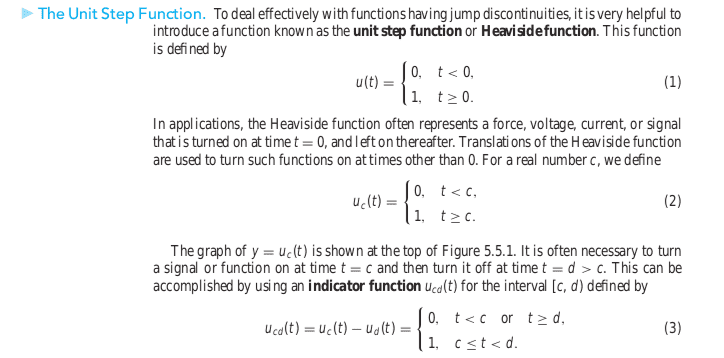# Differential Equation with Summation

## Homework Statement

$$y''+0.1y'+y=1+2\sum_{k=1}^{n}(-1)^{k}u_{k\pi}(t)$$
and quiescent initial conditions.

None.

## The Attempt at a Solution

$$(s^{2}+0.1s+1)Y(s)=\mathcal{L}\{1\}+2\sum_{k=1}^{n}(-1)^{k}\mathcal{L}\big\{ u_{k\pi}(t)\big\}$$

I'm not sure if this step was correct, and how to proceed since the result of that is quite nasty.

Any help would be appreciated, thanks.

LCKurtz
Homework Helper
Gold Member

## Homework Statement

$$y''+0.1y'+y=1+2\sum_{k=1}^{n}(-1)^{k}u_{k\pi}(t)$$
and quiescent initial conditions.

None.

## The Attempt at a Solution

$$(s^{2}+0.1s+1)Y(s)=\mathcal{L}\{1\}+2\sum_{k=1}^{n}(-1)^{k}\mathcal{L}\big\{ u_{k\pi}(t)\big\}$$

I'm not sure if this step was correct, and how to proceed since the result of that is quite nasty.

Any help would be appreciated, thanks.

We aren't mind readers here. What is ## u_{k\pi}(t)##? And what do you get for the transform of the right side?

I'm sorry, I thought it was common notation that $u_{k\pi}(t)$ is the indicator function denoting the unit step function:
$$u_{k\pi}(t)=u_{k}(t)-u_{\pi}(t)=\begin{cases} 0, & t<k\quad\text{or}\quad t\ge\pi\\ 1, & k\le t<\pi \end{cases}$$
As for what I got for the Laplace transform of the right, it is:
$$\frac{1}{s}+2\sum_{k=1}^{n}(-1)^{k}\cdot\frac{e^{-ks}-e^{-\pi s}}{s}$$

LCKurtz
Homework Helper
Gold Member
I'm sorry, I thought it was common notation that $u_{k\pi}(t)$ is the indicator function denoting the unit step function:
$$u_{k\pi}(t)=u_{k}(t)-u_{\pi}(t)=\begin{cases} 0, & t<k\quad\text{or}\quad t\ge\pi\\ 1, & k\le t<\pi \end{cases}$$
As for what I got for the Laplace transform of the right, it is:
$$\frac{1}{s}+2\sum_{k=1}^{n}(-1)^{k}\cdot\frac{e^{-ks}-e^{-\pi s}}{s}$$

For example, if ##k=5## your definition says ##u_{5\pi} = 0\hbox{ if } t<5\hbox{ or }t>\pi##, which is identically zero. Same for any ##k\ge 4##. Something looks fishy to me.

Courtesy of Brannan,The only other possibility is that $c=k\pi$.

Edit: Yes I think thats actually the problem faced here.. I believe it works if I let
$$u_{k\pi}(t)=u(t-k\pi)$$

Last edited:
LCKurtz
Homework Helper
Gold Member
Courtesy of Brannan,The only other possibility is that $c=k\pi$.

Edit: Yes I think thats actually the problem faced here.. I believe it works if I let
$$u_{k\pi}(t)=u(t-k\pi)$$

It shows what a horrible notation that subscripted u is. If you just write u(t-c) when you want it, there is no ambiguity. So apparently you are saying your equation is$$y''+0.1y'+y=1+2\sum_{k=1}^{n}(-1)^{k}u(t-k\pi)$$Is that correct? And when you say "it works", do you mean you see how to finish the question?

Yes, that subscript notation is definitely horrible as it caused me a lot of grief haha.

And yes, by "it works" I meant I solved the DE resulting in the solution of,

$$y(t)=h(t)+2\sum_{k=1}^{n}(-1)^{k}h(t-k\pi)$$

where
$$h(t)=1-e^{-0.05t}\cos(\sqrt{0.9975}t)-\frac{0.05e^{-0.05t}}{\sqrt{0.9975}}\sin(\sqrt{0.9975}t)$$

Thanks for the 'indirect' help!

Last edited:
LCKurtz
Homework Helper
Gold Member
Yes, that subscript notation is definitely horrible as it caused me a lot of grief haha.

And yes, by "it works" I meant I solved the DE resulting in the solution of,

$$y(t)=h(t)+2\sum_{k=1}^{n}(-1)^{k}h(t-k\pi)$$

where
$$1-e^{-0.05t}\cos(\sqrt{0.9975}t)-\frac{0.05e^{-0.05t}}{\sqrt{0.9975}}\sin(\sqrt{0.9975}t)$$

Thanks for the 'indirect' help!

Is that your solution for a particular value of ##n##? Otherwise wouldn't your solution depend on ##n##? Out of curiosity have you graphed your forcing function and solution? What you have is a system being driven for a few cycles by a square wave whose frequency is very near the natural frequency of the system.

Never mind about the n. I see where it is now.

The next part of the question was actually to graph the forcing function and solution on the same set of coordinates. So you are interpreting it correctly.

LCKurtz